# Let f(x,y)=1+x2−cos(5y). Find all critical points and classify them as local maxima, local minima, saddle points, or non...

Let f(x,y)=1+x2−cos(5y). Find all critical points and classify them as local maxima, local minima, saddle points, or none of these.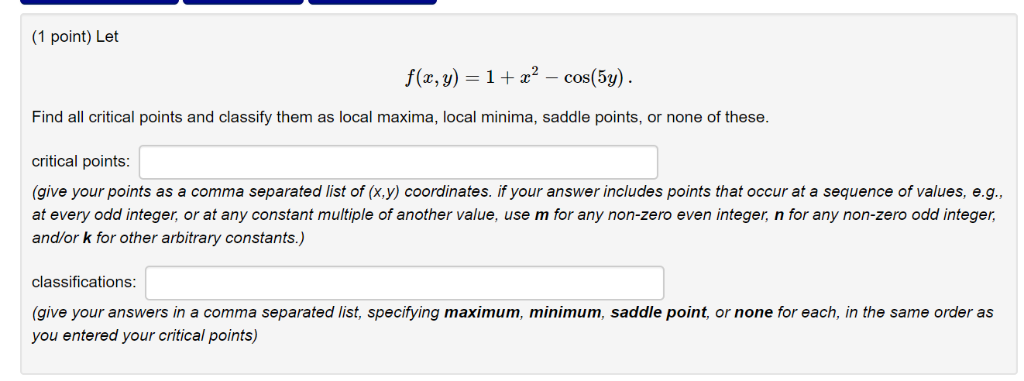(1 point) Let Find all critical points and classify them as local maxima, local minima, saddle points, or none of these. critical points (give your points as a comma separated list of (x,y) coordinates. if your answer includes points that occur at a sequence of values, e.g., at every odd integer, or at any constant multiple of another value, use m for any non-zero even integer, n for any non-zero odd integer, and/or k for other arbitrary constants.) classifications (give your answers in a comma separated list, specifying maximum, minimum, saddle point, or none for each, in the same order as you entered your critical points)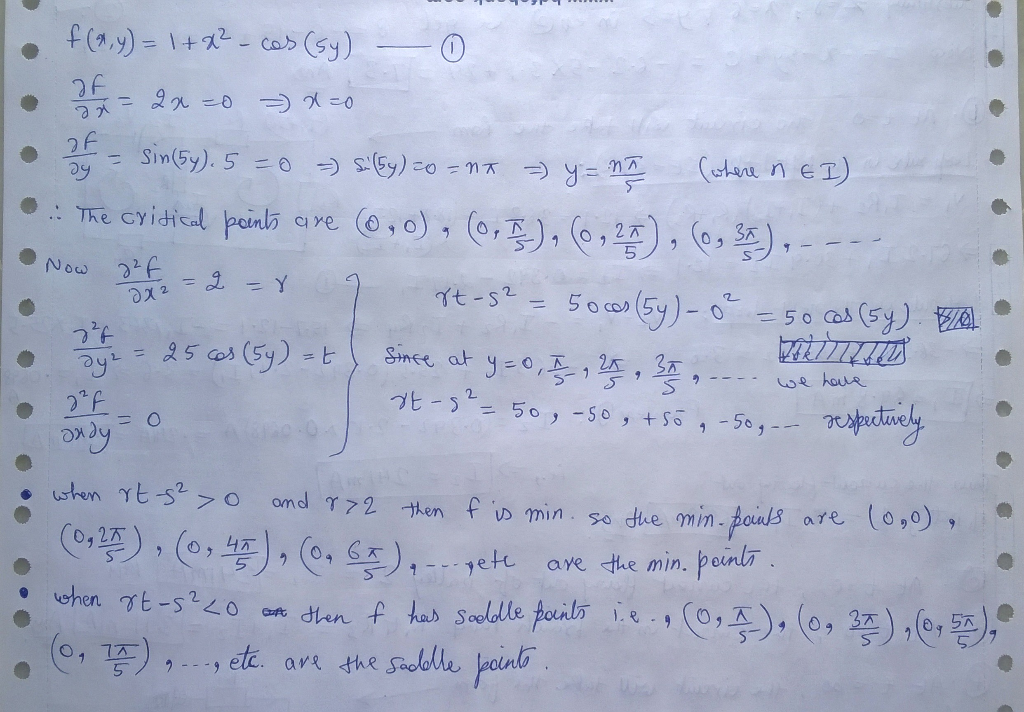##### Add Answer of: Let f(x,y)=1+x2−cos(5y). Find all critical points and classify them as local maxima, local minima, saddle points, or non...
Similar Homework Help Questions
• ### f(r,y)一2 + 2 ry-v2-4r-8y + 9 For Exercises 1-6, find the critical points of the given func- tion and classify them...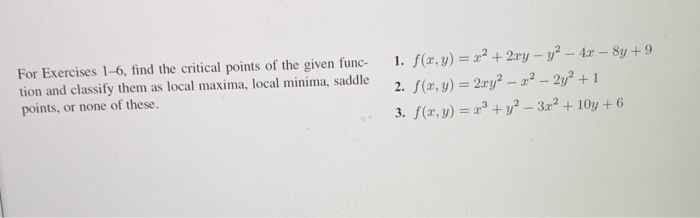f(r,y)一2 + 2 ry-v2-4r-8y + 9 For Exercises 1-6, find the critical points of the given func- tion and classify them as local maxima, local minima, saddle points, or none of these. I. 3. f(z, y) =x3 + y2-3x2 + 10y + 6 f(r,y)一2 + 2 ry-v2-4r-8y + 9 For Exercises 1-6, find the critical points of the given func- tion and classify them as local maxima, local minima, saddle points, or none of these. I. 3. f(z, y) =x3...

• ### Critical Points Question

Find and classify the critical points off(x)=2 x^{3}(7 - x)^4 as local maxima and minima.(Enter your critical points as comma-separated x-value,classification pairs. For example, if you found the critical points x = -2 and x = 3, and that the first was alocal minimum and the second neither a minimum nor a maximum, you should enter (-2,min), (3,neither). Enter none if there are no critical points.)critical points and classifications:

• ### 4. (a) (15 points) Find all the local maxima, local minima, and saddle points of the function f(x, y)ry b) (15 points) Use Lagrange multipliers to find the points on the surface ryz-1 closest to the...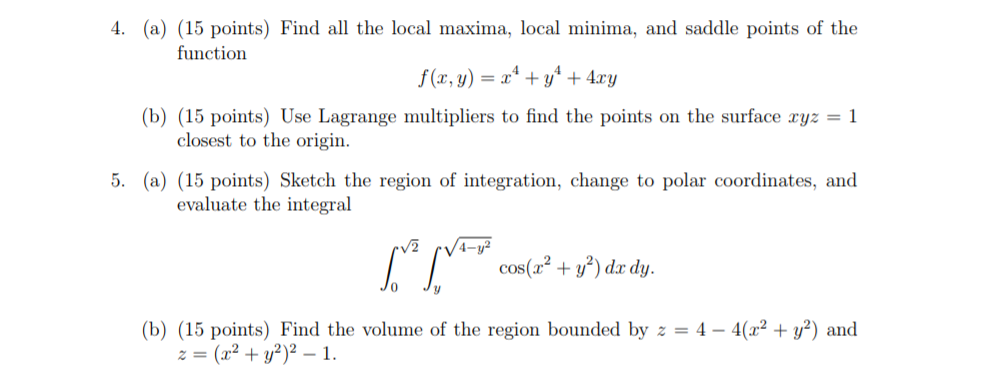4. (a) (15 points) Find all the local maxima, local minima, and saddle points of the function f(x, y)ry b) (15 points) Use Lagrange multipliers to find the points on the surface ryz-1 closest to the origin. polar coordinates, and evaluate the integral cos(a+)d dy. 0 (b) (15 points) Find the volume of the region bounded by z = 4-4(12 + y2) and z=(x2 + y2)2-1. 4. (a) (15 points) Find all the local maxima, local minima, and saddle points...

• ### (17) Consider the function f that is given by f(x, y)-2y +e Find all its critical points and classify each one as a local maximum, local minimum, or saddle point (17) Consider the function f tha...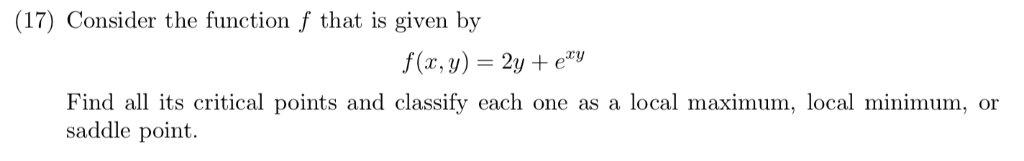(17) Consider the function f that is given by f(x, y)-2y +e Find all its critical points and classify each one as a local maximum, local minimum, or saddle point (17) Consider the function f that is given by f(x, y)-2y +e Find all its critical points and classify each one as a local maximum, local minimum, or saddle point

• ### (A) Find all critical values off. If there are no critical values, enter None. If there...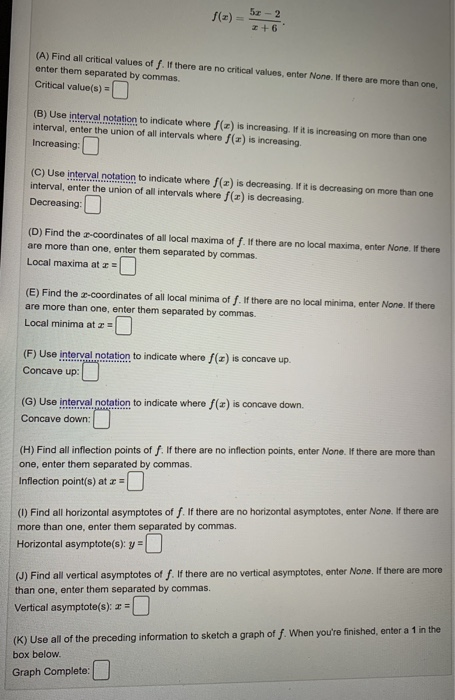(A) Find all critical values off. If there are no critical values, enter None. If there are more than one enter them separated by commas. Critical value(s) = (B) Use interval notation to indicate where f(a) is increasing. If it is increasing on more than one interval, enter the union of all intervals where f(a) is increasing Increasing: (C) Use interval notation to indicate where f(a) is decreasing. If it is decreasing on more than one interval, enter the union...

• ### find the critical points,domain points,and local extreme values for the function.

find the critical points,domain points,and local extreme values for the function.y=-x2-4x+1, x≤1y=-x2+3x-6 x>1a)what is/are the critical point(s) or domain endpoint(s) where f' is undefined? (type an integer or a simplified fraction).x=?b)what is/are the critical point(s) where f' is 0? (type an integer or a simplified fraction,use a comma to separate answers as needed).x=?,?c)from the critical point(s) and domain endpoint(s) ,what is/are the point(s) corresponding to local maxima?(type an ordered pair,simplify your answer.useintegers or fractions for any numbers in the expression.use...

• ### find the critical points,domain points,and local extreme values for the function

find the critical points,domain points,and local extreme values for the function.y=-x2-8x+3, x≤1y=-x2+3x-8 x>1a)what is/are the critical point(s) or domain endpoint(s) where f' is undefined? (type an integer or a simplified fraction).x=?b)what is/are the critical point(s) where f' is 0? (type an integer or a simplified fraction,use a comma to separate answers as needed).x=?,?c)from the critical point(s) and domain endpoint(s) ,what is/are the point(s) corresponding to local maxima?(type an ordered pair,simplify your answer.useintegers or fractions for any numbers in the expression.use...

• ### find the critical points,domain points,and local extreme values for the function.

find the critical points,domain points,and local extreme values for the function.y=-x2-4x+1, x≤1y=-x2+3x-6 x>1a)what is/are the critical point(s) or domain endpoint(s) where f' is undefined? (type an integer or a simplified fraction).x=?b)what is/are the critical point(s) where f' is 0? (type an integer or a simplified fraction,use a comma to separate answers as needed).x=?,?c)from the critical point(s) and domain endpoint(s) ,what is/are the point(s) corresponding to local maxima?(type an ordered pair,simplify your answer.use integersor fractions for any numbers in the expression.use...

• ### find the critical points,domain points,and local extreme values for the function.

find the critical points,domain points,and local extreme values for the function.y=-x2-3x+2,x≤1y=-x2+5x-6x>1a)what is/are the critical point(s) or domain endpoint(s) where f' is undefined? (type an integer or a simplified fraction).x=?b)what is/are the critical point(s) where f' is 0? (type an integer or a simplified fraction,use a comma to separate answers as needed).x=?,?c)from the critical point(s) and domain endpoint(s) ,what is/are the point(s) corresponding to local maxima?(type an ordered pair,simplify your answer.use integersor fractions for any numbers in the expression.use a comma...

• ### Question 5 (25 points) : Use Lagrange multipliers to find all the critical points of the function f (x, y, z)-22on the surface 2 -4y2 722. You do not need to determine whether they are maxima, minima...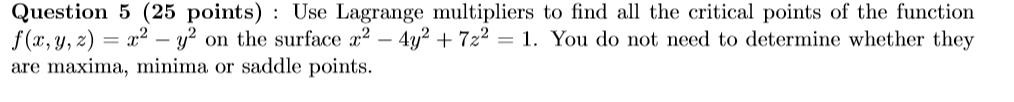Question 5 (25 points) : Use Lagrange multipliers to find all the critical points of the function f (x, y, z)-22on the surface 2 -4y2 722. You do not need to determine whether they are maxima, minima or saddle points. Question 5 (25 points) : Use Lagrange multipliers to find all the critical points of the function f (x, y, z)-22on the surface 2 -4y2 722. You do not need to determine whether they are maxima, minima or saddle points.

Need Online Homework Help?